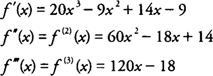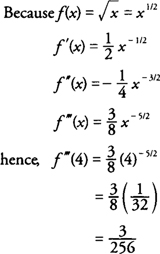## Higher Order Derivatives

Because the derivative of a function y = f( x) is itself a function y′ = f′( x), you can take the derivative of f′( x), which is generally referred to as the second derivative of f(x) and written f“( x) or f 2( x). This differentiation process can be continued to find the third, fourth, and successive derivatives of f( x), which are called higher order derivatives of f( x). Because the “prime” notation for derivatives would eventually become somewhat messy, it is preferable to use the numerical notation f( n )( x) = y( n ) to denote the nth derivative of f( x).

Example 1: Find the first, second, and third derivatives of f( x) = 5 x 4 − 3x 3 + 7x 2 − 9x + 2.Example 2: Find the first, second, and third derivatives of y = sin 2 xExample 3: Find f (3) (4) if.